[Next] [Previous] [Up] [Top]

NUMERICAL METHOD FOR SPECIATION AND FORWARD MODELING

## Application to Reaction and Transport Calculations

The complete set of Newton-Raphson equations that can be included in reaction and transport calculation are derived from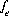,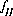,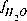,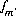,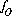,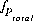,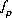,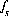,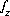,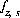,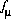, and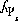. A mole-balance equation for alkalinity can not be included; it is used only in initial solution calculations. All mole-balance equations are for total concentrations of elements, not individual valence states or combinations of individual valence states. The charge-balance equation,, is always used to calculate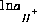. The mole-balance equation on hydrogen,, is always used to calculate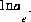. The mole-balance equation on oxygen,, is always used to calculate the mass of water in the system,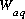. The equationis included if a gas phase is specified and is present at equilibrium. The equationsare included if an exchange assemblage is specified. The equationis included if a surface assemblage is specified. In addition,is included if an implicit diffuse-layer calculation is specified oris included if an explicit diffuse-layer calculation is specified. An equationis included for each pure phase that is present at equilibrium.

It is not known at the beginning of the calculation whether a particular pure phase or a gas phase will be present at equilibrium. Thus, at each iteration, the equation for a phase is included if it has a positive number of moles,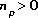, or if the saturation index is calculated to be greater than the target saturation index. If the equation is not included in the matrix, then all coefficients for the unknown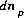in the matrix are set to zero. Similarly, at each iteration, the equation for the sum of partial pressures of gas components in the gas phase is included if the number of moles in the gas phase is greater than a small number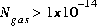, or the sum of the partial pressures of the gas-phase components, as calculated from the activities of aqueous species, is greater than the total pressure. If the equation for the sum of the partial pressures of gas components in the gas phase is not included in the matrix, then all coefficients of the unknown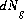are set to zero.

Equationsandare included as optimization equations in the solver. All other equations are included as equality constraints in the solver. In addition, several inequality constraints are included in the solver: (1) the value of the residual of an optimization equation, which is equal to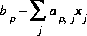, is constrained to be nonnegative, which maintains an estimate of saturation or undersaturation for the mineral, (2) the residual of the optimization equation foris constrained to be nonnegative, which maintains a nonnegative estimate of the total gas pressure, (3) the decrease in the number of moles in the gas phase,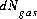, is constrained to be less than the number of moles in the gas phase,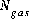, and (4) the decrease in the mass of a pure phase,, is constrained to be less than or equal to the total moles of the phase present,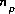.

Initial values for the master unknowns for the aqueous phase are taken from the previous distribution of species for the solution. If mixing of two or more solutions is involved, the initial values are the sums of the values in the solutions, weighted by their mixing factor. If exchangers or surfaces have previously been equilibrated with a solution, initial values are taken from the previous equilibration. If they have not been equilibrated with a solution, the estimates of the master unknowns are the same as those used for initial exchange and initial surface calculations. Initial values for the number of moles of each phase in the pure-phase assemblage and each gas component in the gas phase are set equal to the input values or the values from the last simulation in which they were saved.

For data input to PHREEQC, definition of reaction and transport calculations rely on many of the keyword data blocks. Initial conditions are defined with SOLUTION, EXCHANGE, SURFACE, GAS_PHASE, and EQUILIBRIUM_PHASES keyword data blocks. Reactions are defined with MIX, REACTION, REACTION_TEMPERATURE, and USE keyword data blocks. Transport calculations are specified with the TRANSPORT keyword data block. (See Description of Data Input.)

User's Guide to PHREEQC - 07 MAY 96
[Next] [Previous] [Up] [Top]